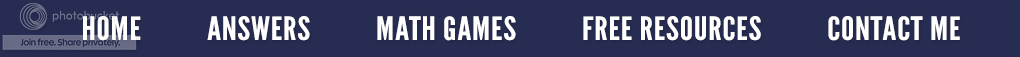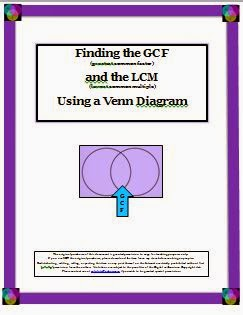### Finding the Greatest Common Factor and Least Common Multiple

The most common method to find the greatest common factor (GCF) is to list all of the factors of each number, then list the common factors and choose the largest one.  Example: Find the GCF of 36 and 54.

1) The factors of 36 are: 1, 2, 3, 4, 6, 9, 12, 18, and 36.

2) The factors of 54 are: 1, 2, 3, 6, 9, 18, 27, and 54.

Therefore, the common factor(s) of 36 and 54 are: 1, 2, 3, 6, 9, 18.  Although the numbers in bold are all common factors of 36 and 54, 18 is the greatest common factor.

To find the lowest common multiple (LCM), students are asked to list all of the factors of the given numbers. Let's say the numbers are 9 and 12.

1) The multiples of 9 are: 9, 18, 27, 36, 45, 54.

2) The multiples of 12 are: 12, 24,  36, 48, 60.

As seen above, the least common multiple for these two numbers is 36.  I have seen this done on a large letter M as illustrated below.

We often instruct our students to first list the prime factors, then multiply the common prime factors to find the GCF. Often times, if just this rule is given, students become lost in the process. Utilizing a visual can achieve an understanding of any concept better than just a rule. A two circle Venn Diagram is such a visual and will allow students to follow the process as well as to understand the connection between each step. For example: Let’s suppose we have the numbers 18 and 12.

1) Using factor trees, the students list all the factors of each number.

2) Now they place all the common factors in the intersection of the two circles. In this case, it would be the numbers 2 and 3.

3) Now the students place the remaining factors in the correct big circle(s).

4) That leaves the 18 with a 3 all by itself in the big circle. The 12 has just a 2 in the big circle.

5) The intersection is the GCF; therefore, multiply 2 × 3 to find the GCF of  6.

6) To find the LCM, multiply the number(s) in the first big circle by the GCF (numbers in the intersection) times the number (s) in the second big circle.

3 × GCF × 2 = 3 × 6 × 2 = 36. The LCM is 36.

This is an effective method to use when teaching how to reduce fractions,Finding GCF and LCM
I have turned this method into a resource for Teachers Pay Teachers. It is 16 pages and begins with defining the words factor, greatest common factor and least common multiple. What a factor tree is and how to construct and use a Venn Diagram as a graphic organizer is shown. Step-by-step examples are given as well as student practice pages. How to use a three circle Venn Diagram when given three different numbers is explained. Two pages of blank pages Venn Diagrams are included for classroom practice. To learn more, just click on the title under the resource cover on your right.

Next week's post - Finding the LCM and GCF for a set of algebraic terms.